# 樹狀數組（BIT）—— 一篇就夠了

## 什麼是 BIT ?

### 起源與介紹

Each integer can be represented as sum of powers of two. In the same way, cumulative frequency can be represented as sum of sets of subfrequencies. In our case, each set contains some successive number of non-overlapping frequencies.

In recognition of the close relationship between the tree traversal algorithms and the binary representation of an element index,the name “binar indexed tree” is proposed for the new structure.

### BIT 的原理

$\begin{array} x\hline 符號 & 解釋 \\ \hline f[i] & 原數組第 i 位的值 \\ tree[i] & \mathrm{BIT}中定義的子序列和數組，tree[i]代表某一序列的和\\ c[i] & 代表 \sum_{k=1}^{k=i} f[i], 即前綴和\\ \hline \end{array}$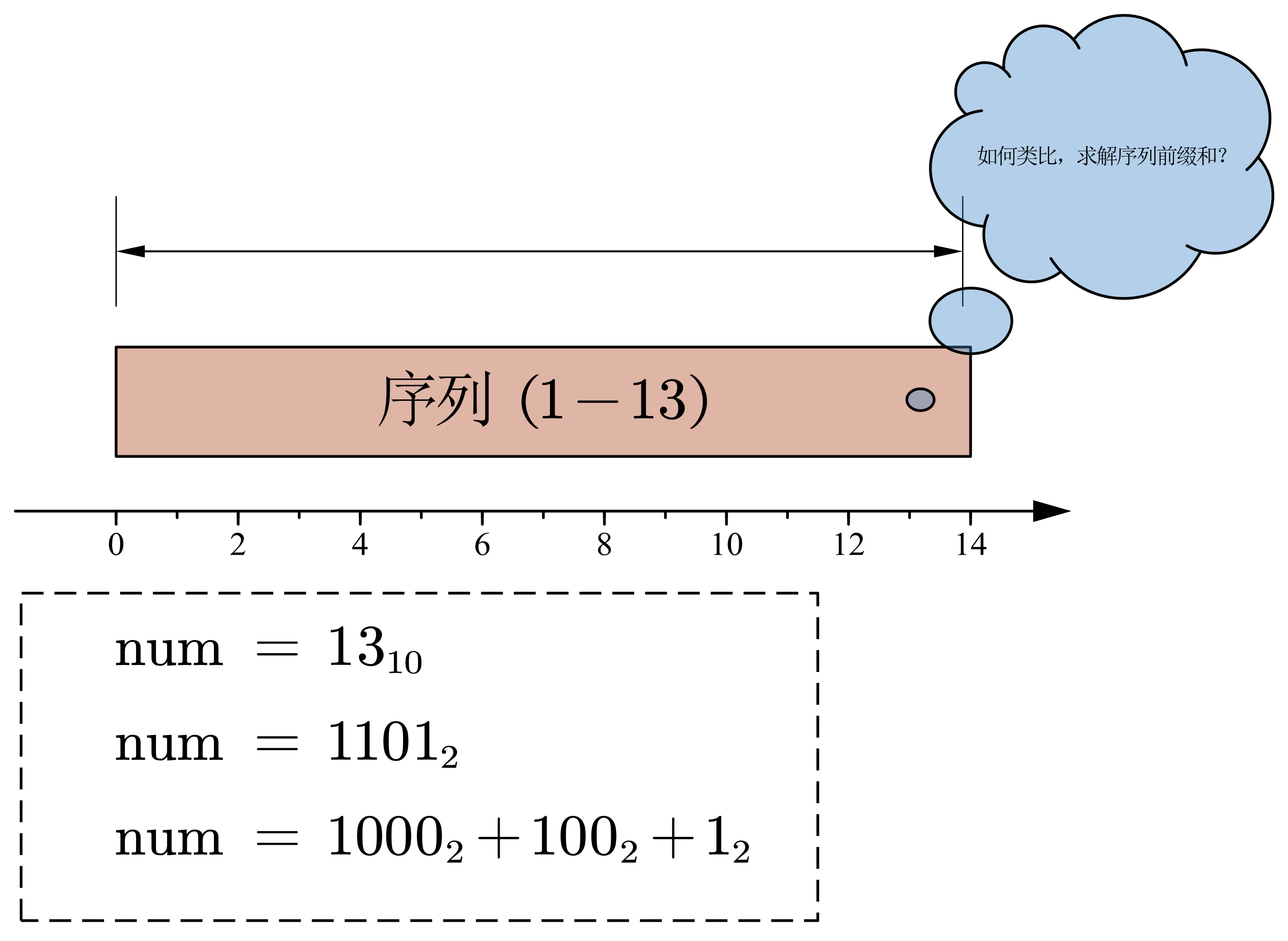• 第一步：思考整數二進位拆分與序列前綴和的類比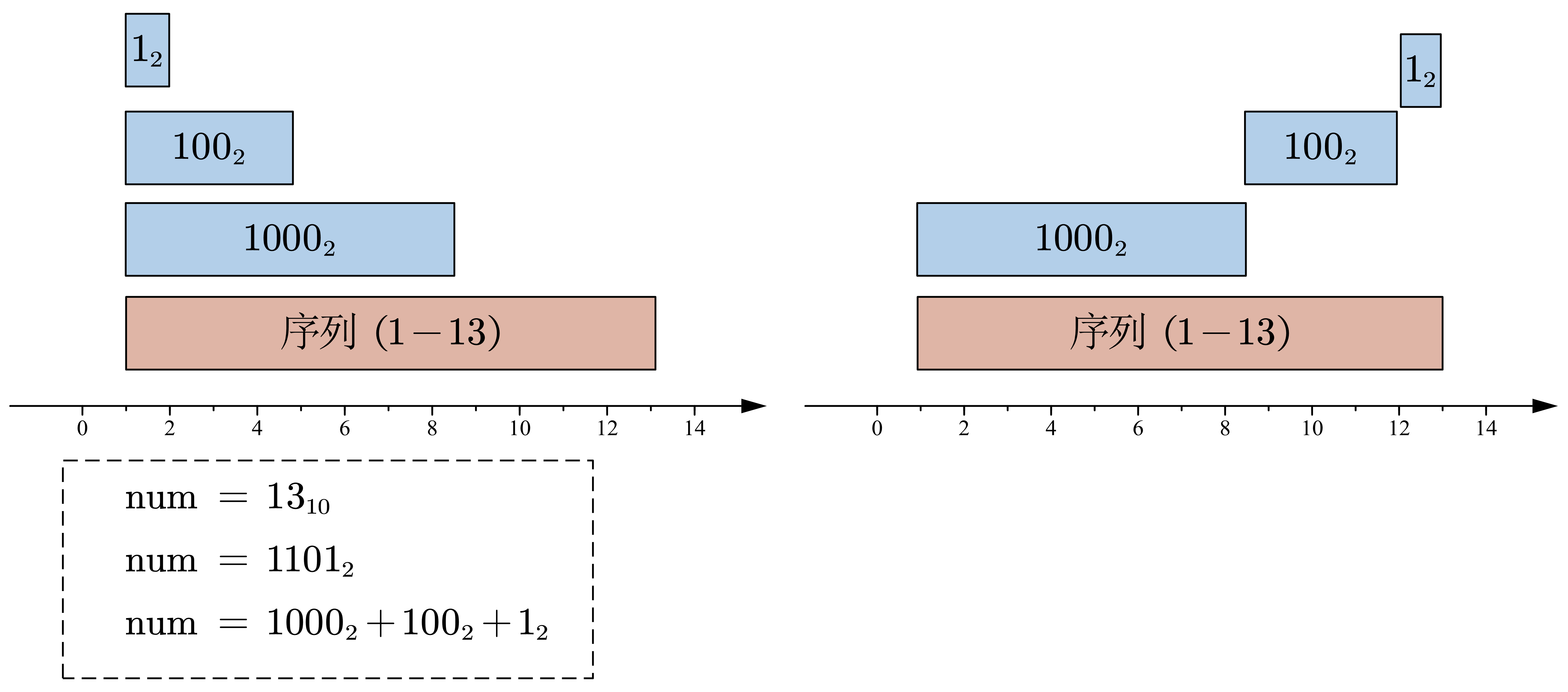\begin{aligned} &num = 13 =1101_2 \\ &子序列_1 = f(f[0001_2]) + f +\cdots + f(f[1000_2]) \quad len(1000_2)\\ &子序列_2 = f(f[1001_2]) + f+\cdots + f(f[1100_2]) \quad len(0100_2)\\ &子序列_3 = f(f[1101_2]) \quad len(0001_2) \end{aligned}

• $$子序列_1$$ 表示的範圍在 $$[0001_1, 1000_2] \rightarrow [0000_2 + 1, 0000_2 + 1000_2]$$
• $$子序列_2$$ 的表示範圍在 $$[1001_2, 1100_2] \rightarrow [1000_2 + 1, 1000_2 + 0100_2]$$
• $$子序列_3$$ 的表示範圍在 $$[1101_2, 1101_2] \rightarrow [1100_2 + 1, 1100_2 + 0001_2]$$

$$[XXX0000_2 + 1, XXX0000_2 + bit000_2]$$ ，換一句話說：假如序列編號在 $$bit$$ 位為1，則其代表的子序列具有如下性質：

1. 子序列的基準量為：$$base = 將二進位編號中bit及其之後所有位置0代表的值\quad eg: num=1101_2,bit=第3位（1-index), 則base = 1000_2$$
2. 子序列的偏移量：$$offset=1<<(bit-1)$$
3. 子序列的下界為：$$lower = base + 1$$
4. 子序列的上界為：$$upper=base+offset$$
5. 子序列包含的元素位：$$tot = offset$$

• $$tree = f \quad \mathrm{lowbit(13) = 1}$$
• $$tree = f + f + f + f \quad \mathrm{lowbit}(12) = 4$$
• $$tree = f + f + \cdots +f \quad \mathrm{lowbit}(8) = 8$$

1. BIT 的原理類比自 Integer 的二進位表示。

2. BIT 對應的數組 $$tree[i] := 子序列 i 的值$$ ，每個 $$tree[i]$$ 控制 $$[i – \mathrm{lowbit(i)}+1, i]$$ 範圍內的$$f[i]$$值。

3. 利用BIT計算 $$c[i]$$ 時，通過類似整數的二進位拆分，將 $$c[i]$$ 拆分為 $$\mathcal{O(\log_2 n)}$$ 個 $$tree[j]$$ 進行求解。求解的流程為不斷累加 $$tree[i]$$ 並置 $i \leftarrow i – \mathrm{lowbit(i)}$

4. 計算流程的偽程式碼：
let ans <- 0
while i > 0:
sub_sum <- tree[i] // 獲取子序列累和
i <- i - lowbit(i) // 更新 i
ans <- ans + sub_sum
return ans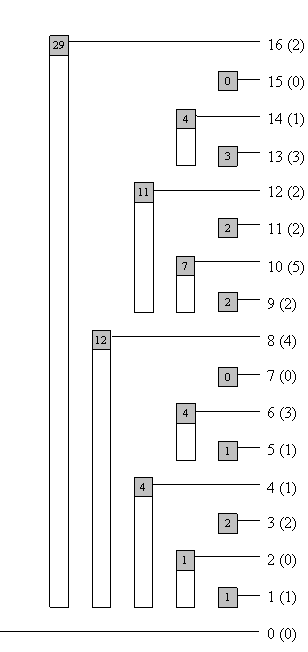### BIT 的詢問，更新操作及其程式碼實現

#### query

$$\mathrm{lowbit}$$ 運算為取數 $$x$$ 的最低位的 1 ,最常用的方法為：$$\mathrm{lowbit(x)= (x \& (-x))}$$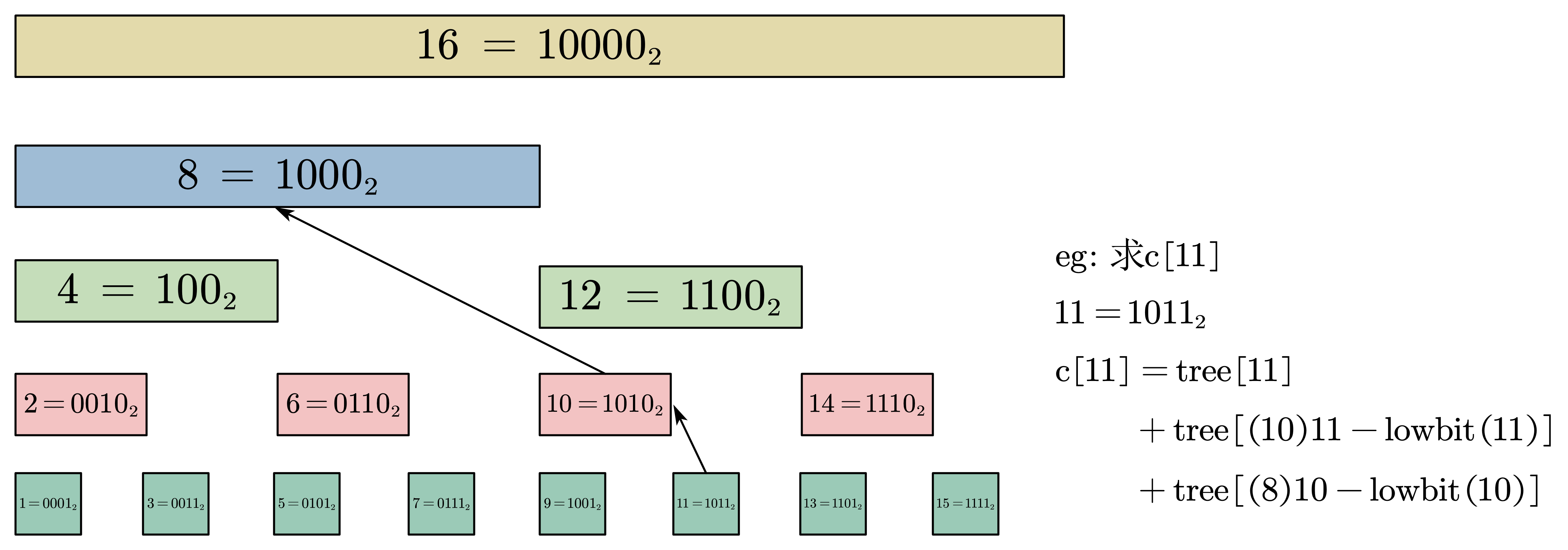C++ 程式碼如下：

T tree[maxn];
template <typename T>
T query(int i){
T res = 0;
while (i > 0){
res += tree[i];
i -= lowbit(i);
}
return res;
}


#### update

update 實際上可以看成 query 的逆過程，簡單來說即是：若要將 $$f[i] += x$$，則從 $$tree[i]$$ 開始不斷向上更新直到達到 BIT 的上界。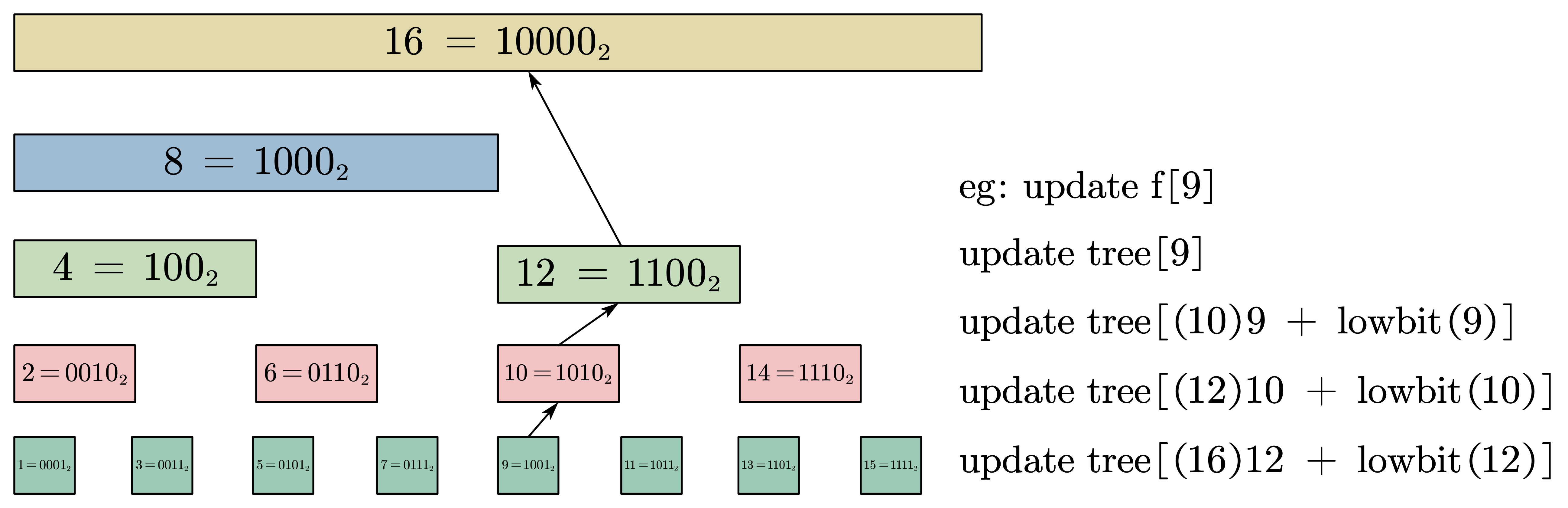C++ 程式碼如下：

int n; // BIT 的大小， BIT index 從 1 開始
T tree[maxn];
template <typename T>
while (i <= n){
tree[i] += x;
i += lowbit(i);
}
}


### 模板

template<typename T>
struct BIT{
#ifndef lowbit
#define lowbit(x) (x & (-x));
#endif
static const int maxn = 1e3+50;
int n;
T t[maxn];

BIT<T> () {}
BIT<T> (int _n): n(_n) { memset(t, 0, sizeof(t)); }
BIT<T> (int _n, T *a): n(_n) {
memset(t, 0, sizeof(t));
/* 從 1 開始 */
for (int i = 1; i <= n; ++ i){
t[i] += a[i];
int j = i + lowbit(i);
if (j <= n) t[j] += t[i];
}
}

while (i <= n){
t[i] += x;
i += lowbit(i);
}
}

/* 1-index */
T sum(int i){
T ans = 0;
while (i > 0){
ans += t[i];
i -= lowbit(i);
}
return ans;
}
/* 1-index [l, r] */
T sum(int i, int j){
return sum(j) - sum(i - 1);
}
/*
href: //mingshan.fun/2019/11/29/binary-indexed-tree/
note:
C[i] --> [i - lowbit(i) + 1, i]
father of i --> i + lowbit(i)
node number of i --> lowbit(i)
*/
};


## BIT 的優缺點，比較與應用場景

### 優缺點

• 程式碼精鍊，實現輕鬆。
• queryupdate 操作時間複雜度都只需要 $$\mathcal{O(\log n)}$$
• 演算法常數小，相比於線段樹更快（lazy tag 也存在影響）。

• 應用場景有限：較為複雜的區間操作無法實現，只能使用線段樹（稍後會講為什麼不能實現）

線段樹與樹狀數組的區別

## 樹狀數組的經典例題及其技巧

### 模板題：單點修改，區間查詢

#### 程式碼：

// 上述模板部分省略
using ll = long long;
const int maxn = 1e6+50;
ll f[maxn];
int main(){
ios::sync_with_stdio(0);
cin.tie(0);

int n; cin >> n;
int q; cin >> q;
for (int i = 1; i <= n; ++ i) cin >> f[i];
BIT<ll> bit(f, n);

for (int i = 0; i < q; ++ i){
int type; cin >> type;
if (type == 1){
int i, x;
cin >> i >> x;
}else {
int l, r;
cin >> l >> r;
cout << bit.sum(l, r) << '\n';
}
}

return 0;
}


### 模板題：區間修改，區間查詢

#### 思路：

1. $$i < l$$

1. $$l <= i <= r$$

1. $$i > r$$

• $$bit_1$$$$l$$ 位置加上 $$-x(l-1)$$，在 $$bit_1$$$$r$$ 位置加上 $$rx$$
• $$bit_2$$$$l$$ 位置加上 $$x$$$$r$$ 位置加上 $$-x$$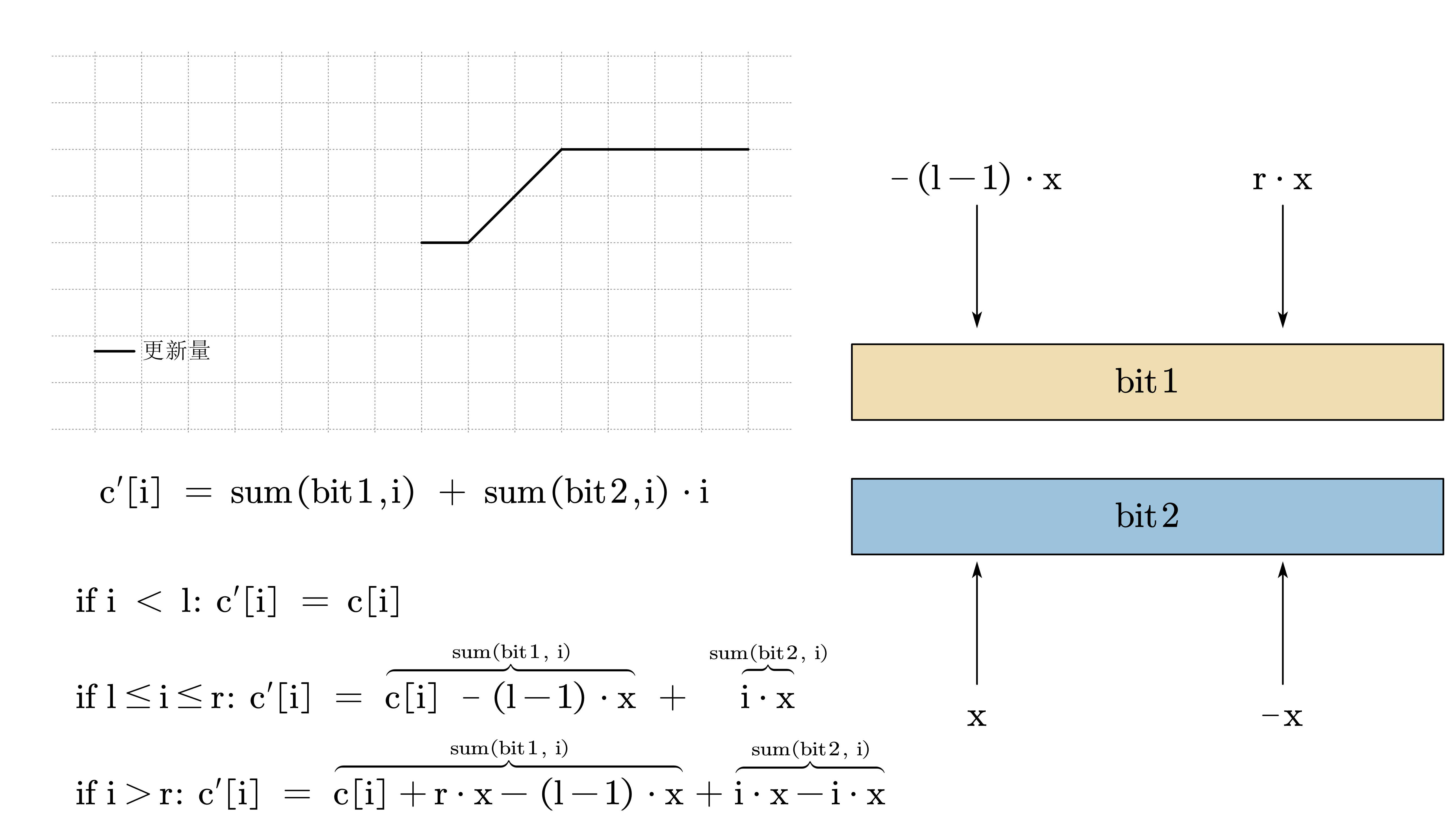#### 程式碼

#include <bits/stdc++.h>
using namespace std;
// 模板程式碼省略
// 這裡做的是單點查詢，但是實現的為區間查詢
using ll = long long;
ll get_sum(BIT<ll> &a, BIT<ll> &b, int l, int r){
auto sum1 = a.sum(r) * r + b.sum(r);
auto sum2 = a.sum(l - 1) * (l - 1) + b.sum(l - 1);
return sum1 - sum2;
}

int n, q;
const int maxn = 1e6 + 50;
ll f[maxn];
int main(){
// ios::sync_with_stdio(0);
// cin.tie(0);

cin >> n >> q;
BIT<ll> bit1, bit2;
for (int i = 1; i <= n; ++ i) cin >> f[i];
bit1.init(n), bit2.init(f, n);

for (int i = 0; i < q; ++ i){
int type; cin >> type;
if (type == 1){
int l, r, x;
cin >> l >> r >> x;
bit2.add(l, (ll) -1 * (l - 1) * x), bit2.add(r + 1, (ll) r * x);
}else {
int i; cin >> i;
cout << get_sum(bit1, bit2, i, i) << '\n';
}
}
return 0;
}


### 逆序對 簡單版

#### 思路

BIT 求解逆序對是非常方便的，在初學時我沒有想到過 BIT 還能用於求解逆序對。在這裡我借逆序對來引出一個小技巧：離散化

BIT 求逆序對的方法非常簡單，逆序對指：i < j and a[i] > a[j]，統計逆序對實際上就是統計在該元素 a[i] 之前有多少元素大於他。

1. 我們順序訪問數組中的每個元素 a[i] ，計算區間 [1, a[i]] 的和，更新答案 ans = i - sum([1, a[i]])
2. 然後，我們更新 BIT 中坐標 a[i] 的值，tree[a[i]] <- tree[a[i]] + 1

eg: [2,1,3,4]
BIT: 0, 0, 0, 0
>2, sum(2) = 0, ans += 0 - sum(2) -> ans = 0
BIT: 0, 1, 0, 0
>1, sum(1) = 0, ans += 1 - sum(1) -> ans = 1
BIT: 1, 1, 0, 0
>3, sum(3) = 2, ans += 2 - sum(3) -> ans = 1
BIT: 1, 1, 1, 0
>4, sum(4) = 3, ans += 3 - sum(4) -> ans = 1


#### 程式碼一

// 僅保留核心程式碼
int reversePairs(vector<int>& nums) {
int n = nums.size();
if (n == 0) return 0;
int mx = *max_element(nums.begin(), nums.end());
BIT<int> bit(mx); // 因為最大隻到最大值的位置
int ans(0);
for (int i = 0; i < n; ++ i){
ans += (i - bit.sum(nums[i]));
}
return ans;
}


#### 程式碼二

#define lb lower_bound
#define all(x) x.begin(), x.end()
const int maxn = 5e4 + 50;
struct node{
int v, id;
}f[maxn]; // 離散化結構體
int arr[maxn];
bool cmp(const node&a, const node &b){
return a.v < b.v;
}
class Solution {
public:
int reversePairs(vector<int>& nums) {
int n = nums.size();
if (n == 0) return 0;
BIT<int> bit(n);

for (int i = 1; i <= n; ++ i){
f[i].v = nums[i - 1], f[i].id = i; // 賦值用於排序
}
sort(f + 1, f + 1 + n, cmp);
int cnt = 1, i = 1;
while (i <= n){
/* 用於去重，當有相同元素時其對應的 cnt 應該相同 */
if (f[i].v == f[i - 1].v || i == 1) arr[f[i].id] = cnt;
else arr[f[i].id] = ++cnt;
++ i;
}

int ans = 0;
for (int i = 0; i < n; ++ i){
int pos = arr[i + 1];
ans += i - bit.sum(pos);
}
return ans;
}
};


通用性:★★

1. 設置結構體 node ，包含屬性 valid ，初始化結構體數組 f 和離散化數組 arr
2. 排序 f ，並從 1 開始遍歷，arr[f[i].id] = i，將 val 值更新為 k-th min 也就是其在元素中按大小排列的編號。

### 逆序對加強版： 翻轉對

#### 思路

id1 = 1, val = 2
2 * id1 = 2, val' = 3


eg: nums = [3, 2, 4, 7]
farr = sort(nums) -> farr = [2, 3, 4, 7]
pos(4) = lower_bound(..., 4) - farr.begin() + 1 = 3



eg: nums = [3, 2, 5, 7]
farr = sort(nums) -> farr = [2, 3, 5, 7]
pos(4) = lower_bound(...,4) - farr.beign() + 1 = 3



#### 程式碼

using vi = vector<int>;
using vl = vector<ll>;
#define complete_unique(x) (x.erase(unique(x.begin(), x.end()), x.end()))
#define lb lower_bound
class Solution {
public:
int reversePairs(vector<int>& nums) {

vl tarr;
for (auto &e: nums){
tarr.push_back(e);
tarr.push_back(2ll * e); // 直接把需要離散化的對應元素加入
}

sort(tarr.begin(), tarr.end());
int n = nums.size();
BIT<int> bit(2 * n); // 注意，因為加入了兩倍的元素，所以對應也要開大一點
int res = 0;

for (int i = 0; i < n; ++ i){
res += i - bit.sum(lb(tarr.begin(), tarr.end(), 2ll * nums[i]) - tarr.begin() + 1);
bit.add(lb(tarr.begin(), tarr.end(), nums[i]) - tarr.begin() + 1, 1);
}
return res;
}
};


通用性:★★★★★

1. 初始化數組 farr ，將元素以及需要尋找的元素都加入其中
2. 二分查找即可。

### 二維BIT：區間查詢，單點修改

#### 程式碼

#include <bits/stdc++.h>
using namespace std;
// 模板程式碼省略
using ll = long long;
int n, m, q;
const int maxn = 5e3 + 50;
BIT<ll> f[maxn]; // 二維BIT

void add(int i, int j, ll x){
while (i <= n){
i += lowbit(i);
}
}
ll sum(int i, int j){
ll res(0);
while (i > 0){
res += f[i].sum(j);
i -= lowbit(i);
}
return res;
}

signed main(){
ios::sync_with_stdio(0);
cin.tie(0);

cin >> n >> m;
for (int i = 1; i <= n; ++ i) f[i] = BIT<ll>(m);

int type;
while (cin >> type){
if (type == 1){
int x, y, k; cin >> x >> y >> k;
}else {
int a, b, c, d; cin >> a >> b >> c >> d;
cout << sum(c, d) - sum(c, b - 1) - sum(a - 1, d) + sum(a - 1, b - 1) << '\n';
}
}

return 0;
}


## 後記

oi-wiki 樹狀數組

Binary indexed tree-樹狀數組